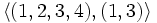# Difference between revisions of "Subgroup structure of symmetric group:S4"

View subgroup structure of particular groups | View other specific information about symmetric group:S4

The symmetric group of degree four has many subgroups.

Note that since$S_4$ is a complete group, every automorphism is inner, so the classification of subgroups upto conjugacy is equivalent to the classification of subgroups upto automorphism. In other words, every subgroup is an automorph-conjugate subgroup.

## Tables for quick information

FACTS TO CHECK AGAINST FOR SUBGROUP STRUCTURE: (finite solvable group)
Lagrange's theorem (order of subgroup times index of subgroup equals order of whole group, so both divide it), |order of quotient group divides order of group (and equals index of corresponding normal subgroup)
Sylow subgroups exist, Sylow implies order-dominating, congruence condition on Sylow numbers|congruence condition on number of subgroups of given prime power order
Hall subgroups exist in finite solvable|Hall implies order-dominating in finite solvable| normal Hall implies permutably complemented, Hall retract implies order-conjugate
MINIMAL, MAXIMAL: minimal normal implies elementary abelian in finite solvable | maximal subgroup has prime power index in finite solvable group

### Table classifying subgroups up to automorphisms

Automorphism class of subgroups Representative Isomorphism class Number of conjugacy classes Size of each conjugacy class Isomorphism class of quotient (if exists) Subnormal depth (if subnormal)
trivial subgroup$\{ () \}$ trivial group 1 1 symmetric group:S4 1
S2 in S4$\{ (), (1,2) \}$ cyclic group:Z2 1 6 -- --
subgroup generated by double transposition in S4$\{ (), (1,2)(3,4) \}$ cyclic group:Z2 1 3 -- 2
A3 in S4$\{ (), (1,2,3), (1,3,2) \}$ cyclic group:Z3 1 4 -- --
Z4 in S4$\langle (1,2,3,4) \rangle$ cyclic group:Z4 1 3 -- --
normal Klein four-subgroup of S4$\{ (), (1,2)(3,4), (1,3)(2,4), (1,4)(2,3) \}$ Klein four-group 1 1 symmetric group:S3 1
non-normal Klein four-subgroups of S4$\langle (1,2), (3,4) \rangle$ Klein four-group 1 3 -- --
S3 in S4$\langle (1,2,3), (1,2) \rangle$ symmetric group:S3 1 4 -- --
D8 in S4$\langle (1,2,3,4), (1,3) \rangle$ dihedral group:D8 1 3 -- --
A4 in S4$\langle (1,2,3), (1,2)(3,4) \rangle$ alternating group:A4 1 1 cyclic group:Z2 1
whole group$\langle (1,2,3,4), (1,2) \rangle$ symmetric group:S4 1 1 trivial group 0

### Table classifying isomorphism types of subgroups

Group name Order Second part of GAP ID (first part is order) Occurrences as subgroup Conjugacy classes of occurrence as subgroup Occurrences as normal subgroup Occurrences as characteristic subgroup
Trivial group 1 1 1 1 1 1
Cyclic group:Z2 2 1 9 2 0 0
Cyclic group:Z3 3 1 4 1 0 0
Cyclic group:Z4 4 1 3 1 0 0
Klein four-group 4 2 4 2 1 1
Symmetric group:S3 6 1 4 1 0 0
Dihedral group:D8 8 3 3 1 0 0
Alternating group:A4 12 3 1 1 1 1
Symmetric group:S4 24 12 1 1 1 1
Total -- -- 30 11 4 4

### Table listing number of subgroups by order

Note that these orders satisfy the congruence condition on number of subgroups of given prime power order: the number of subgroups of order$p^r$ is congruent to$1$ modulo$p$.

Group order Occurrences as subgroup Conjugacy classes of occurrence as subgroup Occurrences as normal subgroup Occurrences as characteristic subgroup
1 1 1 1 1
2 9 2 0 0
3 4 1 0 0
4 7 3 1 1
6 4 1 0 0
8 3 1 0 0
12 1 1 1 1
24 1 1 1 1
Total 30 11 4 4

### Table listing numbers of subgroups by group property

Group property Occurrences as subgroup Conjugacy classes of occurrence as subgroup Occurrences as normal subgroup Occurrences as characteristic subgroup
Cyclic group 17 5 1 1
Abelian group 21 7 2 2
Nilpotent group 24 8 2 2
Solvable group 30 11 4 4

### Table listing numbers of subgroups by subgroup property

Subgroup property Occurences as subgroup Conjugacy classes of occurrences as subgroup Automorphism classes of occurrences as subgroup
Subgroup 30 11 11
Normal subgroup 4 4 4
Characteristic subgroup 4 4 4

## Subgroup structure viewed as symmetric group

### Classification based on partition given by orbit sizes

For any subgroup of$S_4$, the natural action on$\{ 1,2,3,4 \}$ induces a partition of the set$\{ 1,2,3 \}$ into orbits, which in turn induces an unordered integer partition of the number 4. Below, we classify this information for the subgroups.

Conjugacy class of subgroups Size of conjugacy class Induced partition of 4 Direct product of transitive subgroups on each orbit? Illustration with representative
trivial subgroup 1 1 + 1 + 1 + 1 Yes The subgroup fixes each point, so the orbits are singleton subsets.
S2 in S4 6 2 + 1 + 1 Yes$\{ (), (1,2) \}$ has orbits$\{ 1,2 \}, \{ 3 \}, \{ 4 \}$
subgroup generated by double transposition in S4 3 2 + 2 No$\{ (), (1,2)(3,4) \}$ has orbits$\{ 1,2 \}, \{ 3, 4 \}$
A3 in S4 4 3 + 1 Yes$\{ (), (1,2,3), (1,3,2) \}$ has orbits$\{ 1,2,3 \}, \{ 4 \}$
Z4 in S4 3 4 Yes The action is a transitive group action, so only one orbit.
normal Klein four-subgroup of S4 1 4 Yes The action is a transitive group action, so only one orbit.
non-normal Klein four-subgroups of S4 3 2 + 2 Yes$\langle (1,2), (3,4) \rangle$ has orbits$\{ 1,2 \}, \{ 3,4 \}$
S3 in S4 4 3 + 1 Yes$\langle (1,2,3), (1,2) \rangle$ has orbits$\{ 1,2,3 \}, \{ 4 \}$
D8 in S4 3 4 Yes The action is a transitive group action, so only one orbit.
A4 in S4 1 4 Yes The action is a transitive group action, so only one orbit.
whole group 1 4 Yes The action is a transitive group action, so only one orbit.